# SOIL CORROSION ESTIMATION OF ELECTRICAL SUPPORT FOUNDATIONS

Research article
Issue: № 7 (14), 2013
Published:
08.08.2013

Микитинский М.С.

Кандидат технических наук

ОЦЕНКА ГРУНТОВОЙ КОРРОЗИИ ФУНДАМЕНТОВ ЭЛЕКТРИЧЕСКИХ ОПОР

Аннотация

В статье описаны сезонные измерения коррозии фундамента демонтированной опоры линии высокого напряжения. Кривые, полученные методом электрохимической поляризации, обработаны способом линейной регрессии. Результаты этих исследований могут найти применение в мониторинге воздушных линий электропередачи.

Ключевые слова: коррозия, поляризационные кривые, регрессионный анализ.

Mikitinsky M.S.

Ph.D.

SOIL CORROSION ESTIMATION OF ELECTRICAL SUPPORT FOUNDATIONS

Abstract

The polarization method has been applied for corrosion measurements of demounted electrical support legs. Corrosion estimation of the base during drougty period coincides with results of measurements at moist top layer of ground. It allows to use for checks of base the long period without rain. The coefficients of anodic and cathodic polarization are defined by regression analysis. The results of these researches may be use by monitoring of high voltage lines.

Keywords: corrosion, polarization curves, regression analysis.

Introduction

The zinced steel, what placed in concrete, is provided with protection. However presence of defects and cracs in concrete environment allows, that moisture and salts penetrate to surface of steel, causing corrosion. Visual control does not guarantee detection of corrosion, especially below a level of the ground. Opening of foundation ditch and removing of concrete – expensive and labor - consuming procedure.

The known phenomenon of polarization: change of electrical equilibrium potential at influence of external current is used as alternative. For creation of this current was used the circuit EMF 5-10V (constant). Firm “Cormon” (England) produces an instrument TLIM (Tower Leg Integrity Monitor) for mass check of support bases corrosion estimation .

One condition of this method – humidity of soil, where is a foundation. In areas with long droughty period (for example, Israel) there are difficulties for application this method.

Comparison of results in dry and moist soils is purpose of researches, what carried out by us. Also it is necessary to establish dependence of results from duration influence of electric current, since theoretical dependences of electrochemical polarization concern to the certain stage of this process.

The electrical current in limits 0.1- 0.5 mA is created by 6V direct current source, and electrical potential is measured by digital millivoltmeter. A reference electrode Cu/CuSO4 is placed into soil on distance 10 - 15cm from foundation.

Corrosion Current and Polarization Resistance

Connection of corrosion current and electrochemical polarization processes is established in mathematical form is established by M.Stern and A.Geary . Afterwards this dependence has been applied by F.Mansfeld in laboratories researches for comparison of experimental and theoretical polarization curve . It has been allowed to find anodic and cathodic polarization coefficients and using polarization resistance, what is measured, to calculate corrosion current of metal.

In accordance with model, what was accepted in electrochemistry, corrosion current is oxidation current, what arises in anodic zones on metal surfaces. At equilibrium the oxidation current is equal to a reduction current of oxidizer (for example, hydrogen ions or oxygen atoms).

The reduction current arises in cathodic zones of metal surface, what is closed to solution, and is directed opposite to oxidation current. According Tafel Law, at the certain stage there are correlations for oxidation current density ( ia ) and reduction current density ( ic ):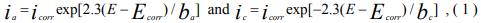where icorr and Ecorr - current density and metal potential, E - potential of metal, what is established at polarization (overvoltage), ba and bc - polarization coefficients.

If corroding metal is connected to positive pole of direct current source in circuit will arise not only anodic current ( ia ), but also opposite cathodic current ( ic ), and the current i in circuit will be equal: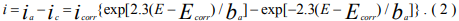)

We’ll consider a case when polarization occurs at the minimal current I, and E - Ecorr→ 0.

We’ll present an exponent by series: exp(x) = 1 + x + 0.5 x2 + . . . Also we’ll limit by first two composed and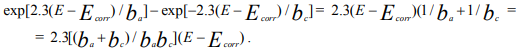Then the equation ( 2 ) will become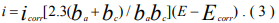From here the density of corrosion current will be expressed by Stern and Geary formula: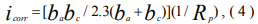where Rp = lim (E - Ecorr)/i , if (E - Ecorr) → 0, is called “polarization resistance”.

Having substitute the formula ( 4 ) in expression ( 2 ), we’ll receive Mansfeld equation: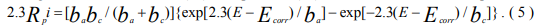The equation ( 5 ) was used in laboratory researches of iron corrosion in H2SO4 solution.

In such experiments i – the density of external current (in mA/sm2) and Rp – polarization resistance (in Ω-sm2) were represented as accessible to supervision constance. At research of underground corrosion of the ferroconcrete bases the area of metal surface is not supervised. In this case it is necessary to pass to integral parameters I and rp , according to relations:

I = iS and rp = Rp/S , ( 6 )

where S – area of all surface of corrosion damages, I – external current what is measured by milliampermeter (in mA), rp – polarization resistance at this current (in Ω).

After substitution from expressions ( 6 ) to left part of equation ( 5 ) we’ll have: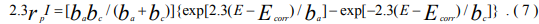Anodic Polarization of Electrical Support Foundation

Measurements were carried out in Israel last months rainy (May, 2004) and droughty (November, 2003) periods. At dry ground the contact place of reference electrode with soil was exposed to humidifying prior to measurements beginning and during all supervision time. The external electric current was adjusted from 0.15 mA up to 0.50 mA through 0.05 mA. Electrical potential of steel armature in relation to surrounding ground was fixed with accuracy 1mV.

As can see from diagrams (Fig.1 and Fig.2), polarization curves are closed very much, exception at dry ground, although this case polarization curves after t = 1.0 and t = 2.0 hours are closed to curve at t = 0 hour too.

Regression Analysis for Coefficients ba and bc

The equation ( 7 ) is represented as regression if to regard his left part as experimental dependence of current I from potential change (E - Ecorr). Then right part of this equation represents theoretical dependence for the same variable (E - Ecorr). Mansfeld entered variables w and x, what connected with required polarization coefficients :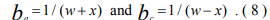The linear regression is possible if intermediate parameter w is expressed by form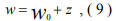where w0 - some arbitrary significance, and z << w0 is small parameter. Finally nonlinear equation ( 7 ) has been presented by linear form: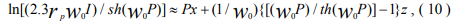where P = 2.3(E - Ecorr), sh(w0P) and th(w0P) – hyperbolic functions.

The equation system ( 10 ) with size n (number measurements) is processed by least squares method, and variables x and z are defined from corresponding normal equations. This procedure was realized by computer [4,5].

For definition of polarization coefficients ba and bc are taken the experimental data, corresponding to polarization during t = 2 hours. Besides by those calculations, significances of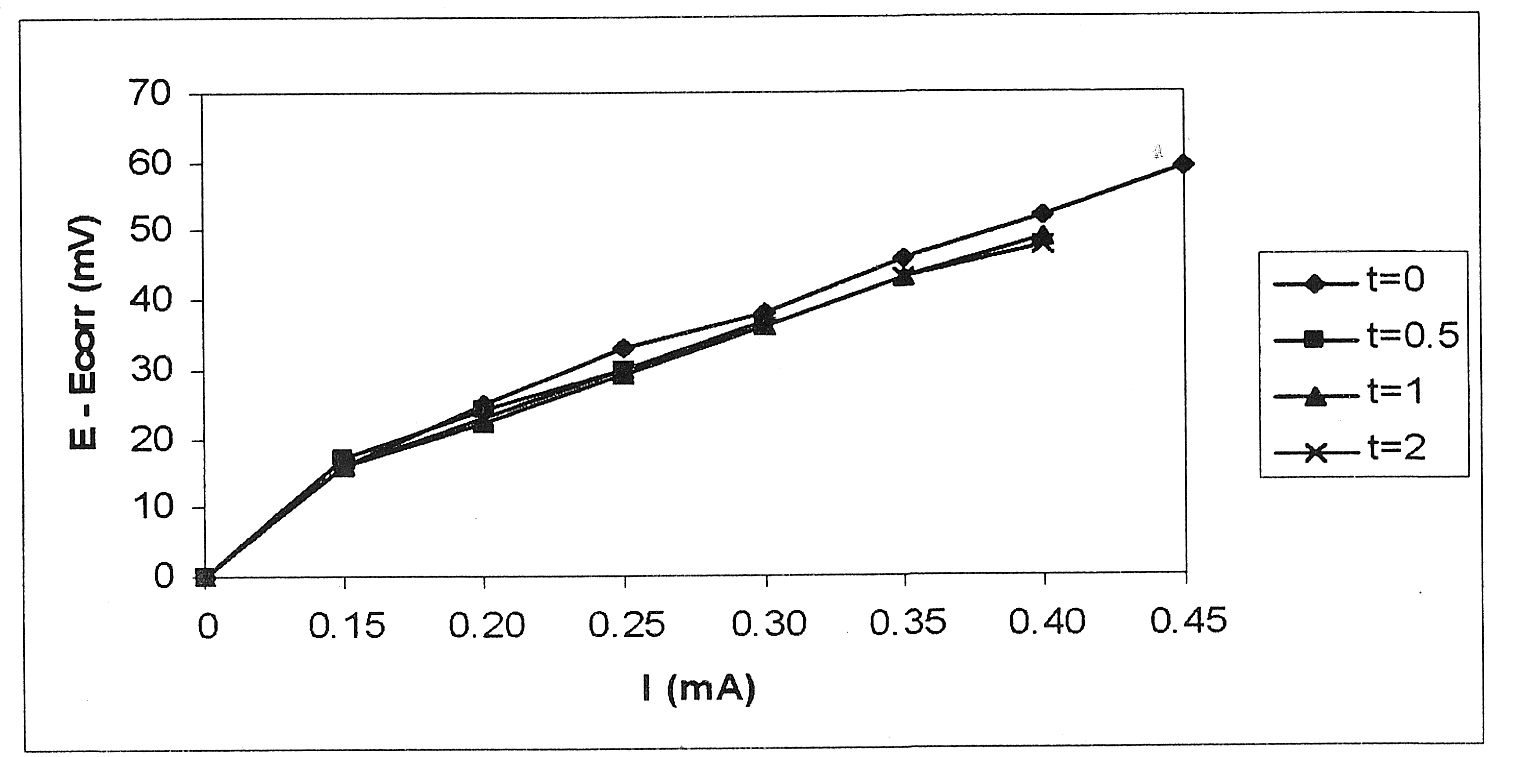Fig.1 - Polarization Curves ( moist Soil )Fig.2 – Polarization Curves ( dry Soil )

variable w from range what specified in Tab.1, are used. Finally w0, bringing to minimal value z/w0, is picked out. In the same table there are significances of polarization coefficients what we have found.

Table 1 - Calculation Data

 soil w W0 mV z/w0 % ba mV bc mV moist 0.0030 - 0.0043 0.0041 - 0.5 386 179 dry 0.0015 - 0.0035 0.0033 +1.0 439 228

Corrosion current Icorr is calculated under formula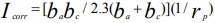what is followed from formula ( 4 ). Besides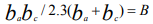is marked (Tab. 2).

Table 2 - Comparison of Results

 soil Icorr mA rp Ω B mV moist 0.5 107 53 dry 0.5 140 65

The comparison result of corrosion currents gives the reason to consider, that top layers of ground, which are exposed atmospheric precipitation, do not exert determining influence on the corrosion deterioration of reinforcing foundations. However such parameters as polarization resistance rp and corrosion factor B can differ for dry soil and moist soil.

Conclusions

The ferroconcrete base was exposed to polarization by direct current 0.15mA during two hours. The polarization curves, what have received after one hour and two hour practically coincide with curve what have received at initial moment of polarization.

The measurements of polarization resistance and coefficients can be made either in moist ground or dry ground if contact place of reference electrode with ground is exposed to sufficient humidity.

Corrosion current what was determined as result of measurement and calculation has the same value in moist and dry soil. This means that corrosion process leading to deterioration of the base affects deep soil layers and no layers what close surface of ground.